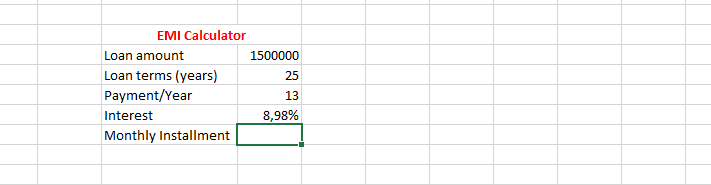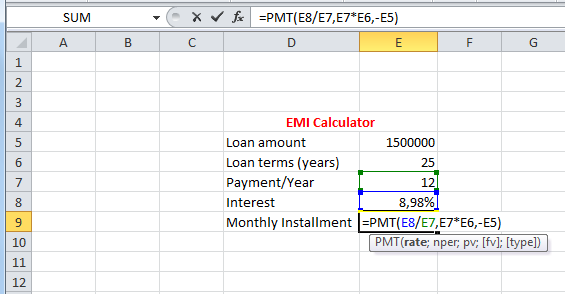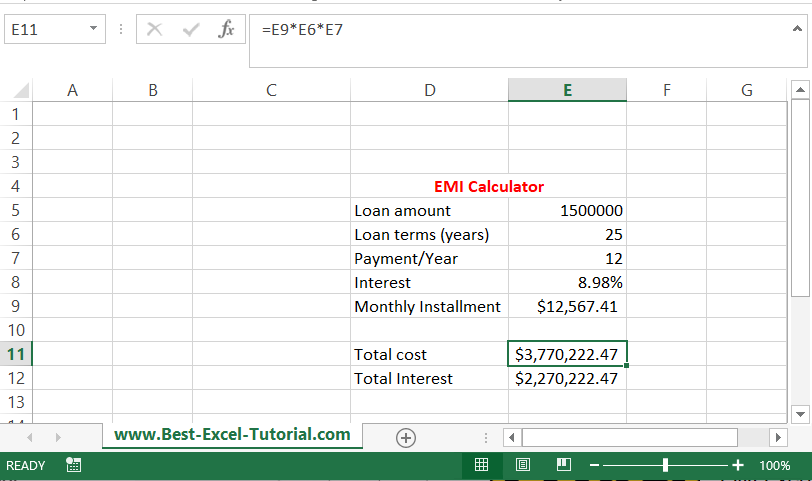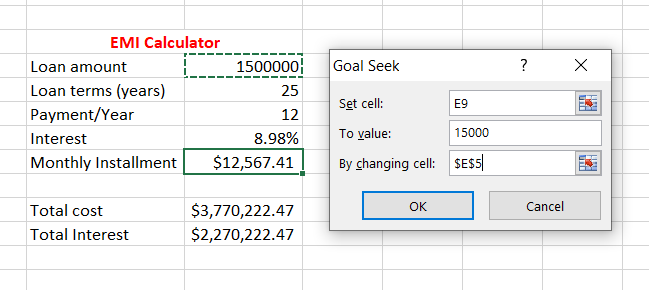## How to calculate EMI?

We are going to learn how to calculate the EMI, with these simple steps. The EMI is Equated Monthly Installment. It is easy to calculate EMI in Excel.Layout the information you already know, without EMI calculation.Click on the column that is beside the monthly installment, and type in

=PMT(rate,nper,pv,[fv],[type])Formula here is =PMT(E8/E7,E7*E6,-E5)

Note:

• The rate is interest divided with payment/year,
• nper is loan terms multiplied by count of payments
• pv was the loan amount with a minus symbol in front. The minus symbol means that this is the money you own.This is the result. Your monthly installment will be \$12 567.41. This is the calculated EMI for \$1,5m for 25 years with the yearly interest of 8,98%.

## How to calculate total loan cost and total interest based on EMI?

But what is the total cost of such loan? Let’s calculate total loan costs and total interest in such situation.Total cost is EMI * years * months. The formula is =E9*E6*E5 in my example.

Total interest is just total cost – loan amount (=E11-E5).

Total cost of such loaned would be over \$3,77m and total interest over \$2,27m.

## How to calculate loan amount based on given EMI?

This is how to calculate EMI with Excel. What if you know your target EMI and would like to know the loan amount you will be able to borrow.

Go to Ribbon to the Data tab. Click What-If Analysis and choose Goal Seek.New dialog window appears. Let’s assume can’t afford \$15 000 monthly installment. Goal Seek parameters will be:

• Set cell – the cell with your EMI (E9 here)
• To value – we agreed 15 000.
• By changing cell – the cell with the loan amount (E5 here).New Loan amount is calculated.With given loan conditions and assuming \$15 000 of your EMI you can borrow \$1 790 345.28 of loan.

In this lesson you learned:

• How to calculate EMI?
• How to calculate total amount?
• How to calculate total loan costs and total interest cost?

## Template

```Further reading: